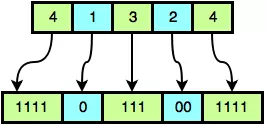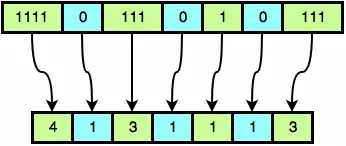# What's Next?

## 题目

### What’s Next?

Johnny is playing with a large binary number, B. The number is so large that it needs to be compressed into an array of integers, A, where the values in even indices `(0, 2, 4,...)` represent some number of consecutive `1` bits and the values in odd indices `(1, 3, 5,...)` represent some number of consecutive `0` bits in alternating substrings of B.

For example, suppose we have array A = `{4, 1, 3, 2, 4}`. A0 represents `"1111"`, A1 represents `"0"`, A2 represents `"111"`, A3 represents `"00"`, and A4 represents `"1111"`. The number of consecutive binary characters in ith the substring of B corresponds to integer Ai, as shown in this diagram:When we assemble the sequential alternating sequences of `1`‘s and `0`‘s, we get B = `11110111001111`.

We define setCount(B) to be the number of `1`‘s in a binary number, B. Johnny wants to find a binary number, D, that is the smallest binary number > B where setCount(B) = setCount(D). He then wants to compress D into an array of integers, C (in the same way that integer array A contains the compressed form of binary string B).

Johnny isn’t sure how to solve the problem. Given array A, find integer array C and print its length on a new line. Then print the elements of array C as a single line of space-separated integers.

#### Input Format

The first line contains a single positive integer, T, denoting the number of test cases. Each of the 2T subsequent lines describes a test case over 2 lines:

1. The first line contains a single positive integer, , denoting the length of array A.
2. The second line contains positive space-separated integers describing the respective elements in integer array A (i.e., A0, A1,…, An-1).

#### Constraints

• 1 T 100
• 1 n 10

• For a score, .
• For a score, .

#### Output Format

For each test case, print the following lines:

1. Print the length of integer array (the array representing the compressed form of binary integer ) on a new line.
2. Print each element of as a single line of space-separated integers. It is guaranteed that a solution exists.

#### Explanation

A = `{4, 1, 3, 2, 4}`, which expands to B = `11110111001111`. We then find setCount(B) = `11`. The smallest binary number > B which also has eleven `1`‘s is D = `11110111010111`. This can be reduced to the integer array C = `{4, 1, 3, 1, 1, 1, 3}`. This is demonstrated by the following figure:Having found C, we print its length (`7`) as our first line of output, followed by the space-separated elements in C as our second line of output.

## 思路

• 最后跟的全是`1`：最简单的就是最后全是`1`，只需要将第一个`1`和前面的`0`互换位置就可以，`100111` => `101011`

• 最后跟的全是`0`：最后全`0`的时候，需要将前面的全`1`的第一个`1`和左边的`0`呼唤，然后将剩下所有的`1`和右侧所有的`0`互换位置。